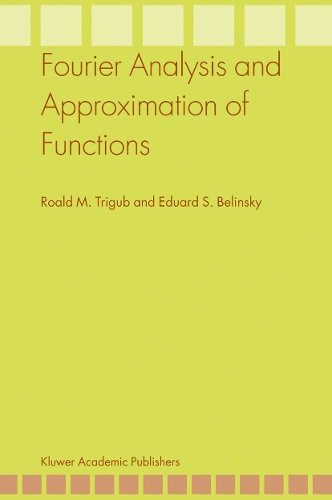•# Fourier Analysis and Approximation of Functions (Paperback)

(author), (author)
£90.00
Paperback 586 Pages / Published: 06/12/2010
• We can order this

Usually dispatched within 3 weeks

In Fourier Analysis and Approximation of Functions basics of classical Fourier Analysis are given as well as those of approximation by polynomials, splines and entire functions of exponential type.
In Chapter 1 which has an introductory nature, theorems on convergence, in that or another sense, of integral operators are given. In Chapter 2 basic properties of simple and multiple Fourier series are discussed, while in Chapter 3 those of Fourier integrals are studied.
The first three chapters as well as partially Chapter 4 and classical Wiener, Bochner, Bernstein, Khintchin, and Beurling theorems in Chapter 6 might be interesting and available to all familiar with fundamentals of integration theory and elements of Complex Analysis and Operator Theory. Applied mathematicians interested in harmonic analysis and/or numerical methods based on ideas of Approximation Theory are among them.
In Chapters 6-11 very recent results are sometimes given in certain directions. Many of these results have never appeared as a book or certain consistent part of a book and can be found only in periodics; looking for them in numerous journals might be quite onerous, thus this book may work as a reference source.
The methods used in the book are those of classical analysis, Fourier Analysis in finite-dimensional Euclidean space Diophantine Analysis, and random choice.

Publisher: Springer
ISBN: 9789048166411
Number of pages: 586
Weight: 908 g
Dimensions: 235 x 155 x 30 mm
Edition: Softcover reprint of hardcover 1st ed. 2004

MEDIA REVIEWS

From the reviews:

"The motivation for writing this extensive monograph of over 500 pages is to compile the basic and more current results in Fourier analysis that are particularly useful for solving certain approximation problems. ... This is a very well written monograph with very rich content. It is an excellent reference source for researchers in approximation theory, and is also suitable for adoption as textbook for a graduate course ... ." (Charles K. Chui, Mathematical Reviews, Issue 2005 k)

"Part of the book is classical approximation theory and classical Fourier analysis (series and integrals in one and more variables) with the emphasis on approximation theory. ... each chapter is concluded by a rather extensive section 'Further problems and theorems' which introduces extended or related results as exercises, often suggesting methods for the solutions, and a guide to the literature. ... When used as a textbook, it will be a ... source of inspiration for a researcher in approximation theory or harmonic analysis." (Adhemar Bultheel, Bulletin of the Belgian Mathematical Society, Vol. 15 (1), 2008)